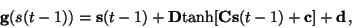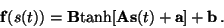Next: Improved approximation of the Up: Unsupervised Learning of Nonlinear Previous: Predictable factors and state-space

# Model and learning algorithm

The functional form and learning algorithm for the nonlinear dynamic factor analysis (NDFA) model proposed in this report are very close to the ones introduced for NLFA in : the mappings f and g are modelled by MLP networks and a gradient based learning algorithm is used for iteratively updating the approximation of the posterior probability of the unknown variables. There are some modifications, however, which take into account the fact that the mapping g models the dynamics of the factors.

First of all, it can be expected that the values of the factors s(t) are close to the values at the previous time step s(t-1). This prior knowledge can be taken into account by using the MLP network to model only the change in factors instead of trying to learn the whole mapping from s(t-1) to s(t). The functional form of the dynamic mapping gis thus(3)

while the observation mapping is(4)

It should be noted that the MLP network modelling the dynamics can learn to overrule the term s(t-1) if it turns out that the value of the factor at time t-1 does not bear information about the value of the factor at time t.Next: Improved approximation of the Up: Unsupervised Learning of Nonlinear Previous: Predictable factors and state-space
Harri Valpola
2000-10-17